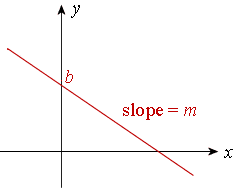# RD Sharma Solutions Class 12 Chapter 28 Straight Line In Space

## RD Sharma Solutions for Class 12 Maths Chapter 28 – Free PDF Download

The RD Sharma Solutions for Class 12 Maths Chapter 28 Straight Line in Space is provided here. Students can utilise these RD Sharma Solutions for Class 12 as a reference while solving the exercise questions of RD Sharma Class 12 Maths. Detailed solutions help students strengthen their conceptual knowledge and problem-solving skills.

RD Sharma Solutions is a valuable resource for students preparing for the Class 12 Maths board exam and other competitive exams like JEE Mains. Referring to these RD Sharma Solutions created by subject experts at BYJU’S will benefit students while solving the RD Sharma Textbook questions. To help students fetch high marks, these solutions are curated according to the latest CBSE guidelines and marking schemes.

### Highlights of RD Sharma Class 12 Solutions Chapter 28

A line extending on both sides to infinity without any curves is called a straight line. It is an object in geometry that is characterised as zero-width and extends in opposite directions without any end. A straight line was the first topic to be explained by Euclid – the founder of Geometry.The equation of straight lines can be expressed in the form:y = mx + b

Where y is the intercept

x is how far along

m is the slope or the gradient.

b is the intercept of y.

For example:

The line y = 3x + 5 has

Slope m = 3 and y-intercept b = 5.

Practise problems on Straight lines through solved RD Sharma Solutions to build a better understanding of the topic.

 Straight Line in Space Class 12 RD Sharma Exercises Straight Line in Space Exercise 28.1 Straight Line in Space Exercise 28.2 Straight Line in Space Exercise 28.3 Straight Line in Space Exercise 28.4 Straight Line in Space Exercise 28.5